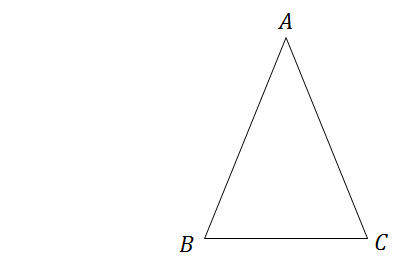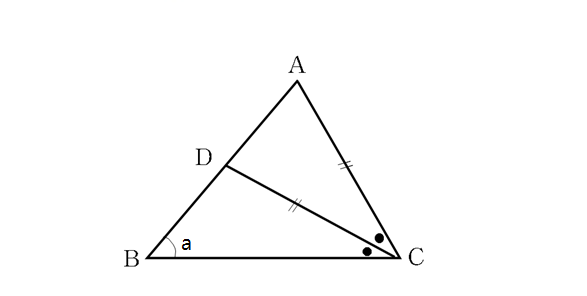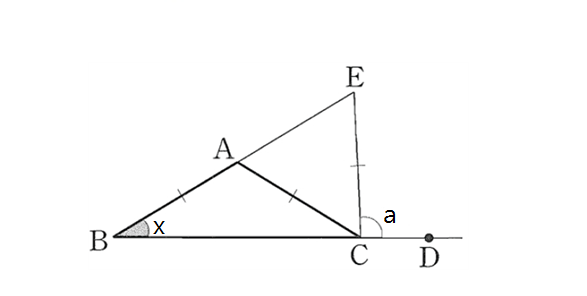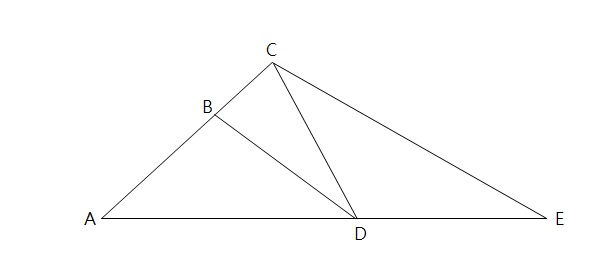Geometry

# Properties of Isosceles Triangles$\triangle ABC$ is an isosceles triangle such that the lengths of $\overline{AB}$ and $\overline{AC}$ are equal. If $\angle BAC=78 ^\circ ,$ what is $\angle ABC$ in degrees?In the above diagram, $\triangle ACD$ is an isosceles triangle with the length of $\overline{CA}$ equal to the length of $\overline{CD}.$ If $\overline{CD}$ bisects $\angle ACB$ and $\angle ABC =a= 66^{\circ},$ what is three times $\angle ACD$ in degrees?In the above diagram, $\angle DCE=a=99^\circ, \lvert \overline{AB}\rvert =\lvert \overline{AC}\rvert=\lvert \overline{CE}\rvert,$ and $\overline{BE}$ and $\overline{BD}$ are both straight lines. What is the value of $\angle ABC(=x)$ in degrees?

Note: The above diagram is not drawn to scale.In the above diagram, $\angle{BAD} = 22^{\circ}, \overline{AB}=\overline{BD}=\overline{CD}=\overline{DE}.$ What is $\angle{CEA}?$

Note: The above diagram is not drawn to scale.

Which of the following does NOT sufficient to indicate an isosceles triangle?

×